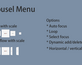Unity Carousel Menu · By MisoStudio

# position error

A topic by chelaton created May 09, 2020 Views: 182 Replies: 18
Viewing posts 1 to 3

rt.position = m_final; hi this part change position of other buttons to 110000 instead 110 .. any help?

Developer

Hi, can you elaborate what you have done to cause this issue, thanks

Hi, thanks for replay. If your Canvas scale is different than 1,1,1 .. mine is 0.001, 0.001, 0.001 and x positions of buttons are 110000, -110000, 220000, -220000.

Developer

Hi, in that case, I think you can  do this:

RectTransform _canvasRect;

m_final = new Vector3
(
m_final.x * _canvasRect.localScale.x,
m_final.y * _canvasRect.localScale.y,
m_final.z * _canvasRect.localScale.z
);

Tried and not working. Probably its not so easy.  Tried to change your demo  canvas and result is the same.

Developer

Is this the issue you are having?

I'm not sure. But you didn't change canvas scale too much .. only 0.5 . change it to 0.001, 0.001, 0.001 and x positions of buttons will be 110000, -110000, 220000, -220000.

My screen from your demo. I changed Canvas scale ... run the game ..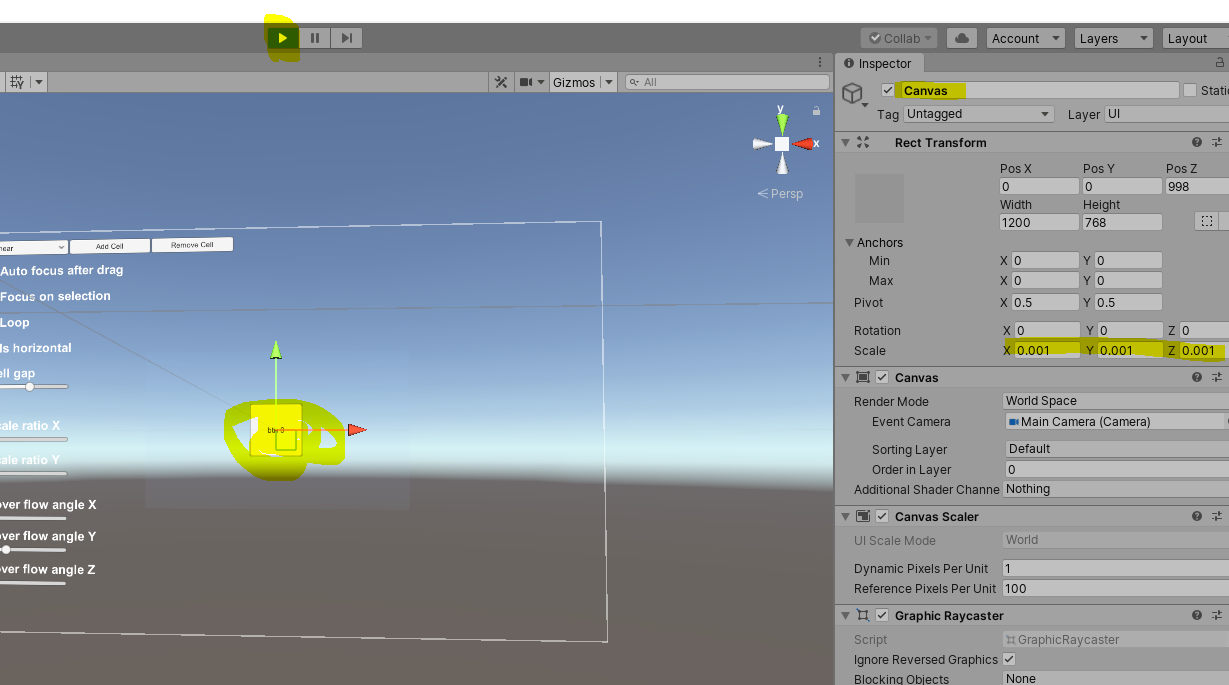Here is the button 1 position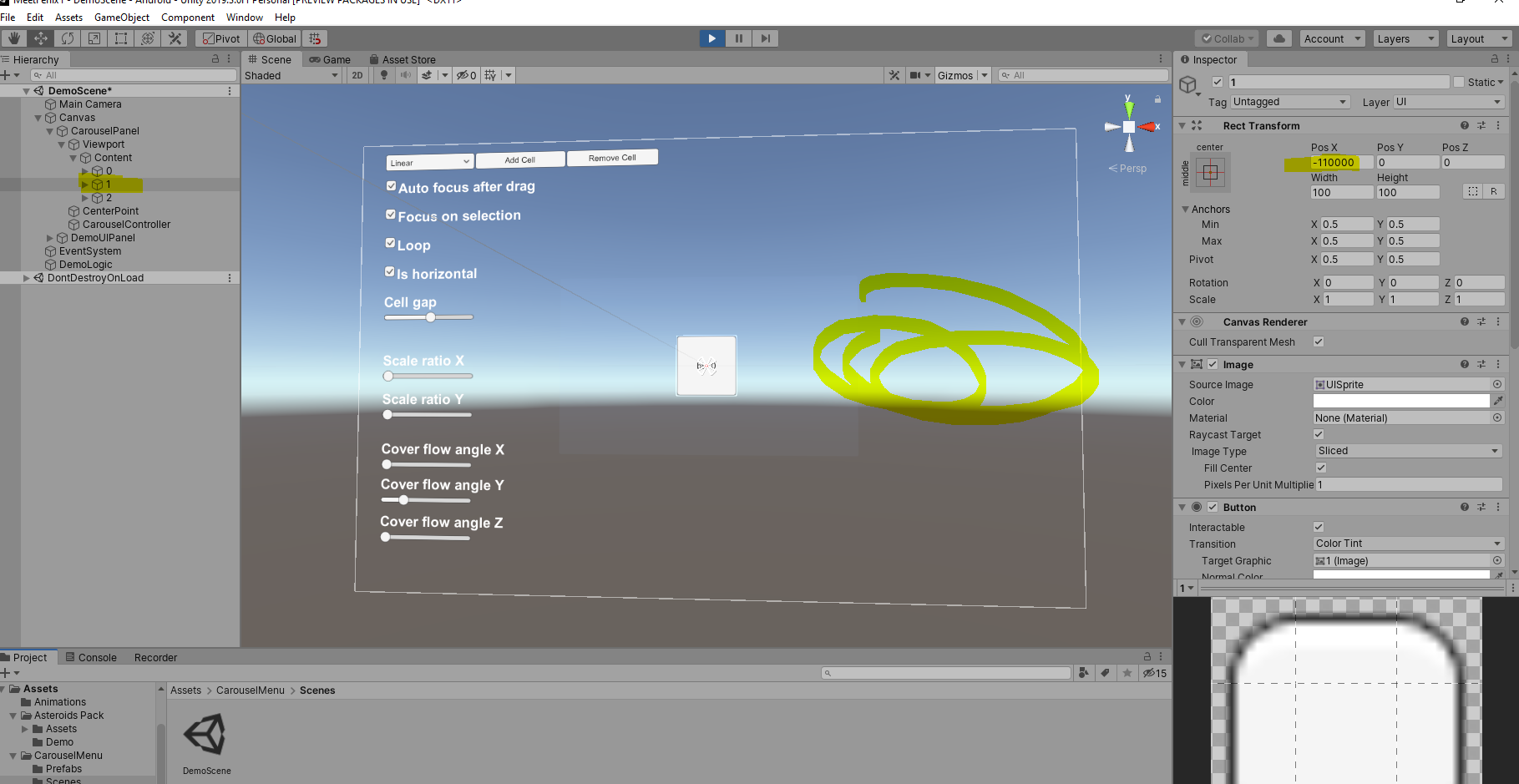Here is button 2 position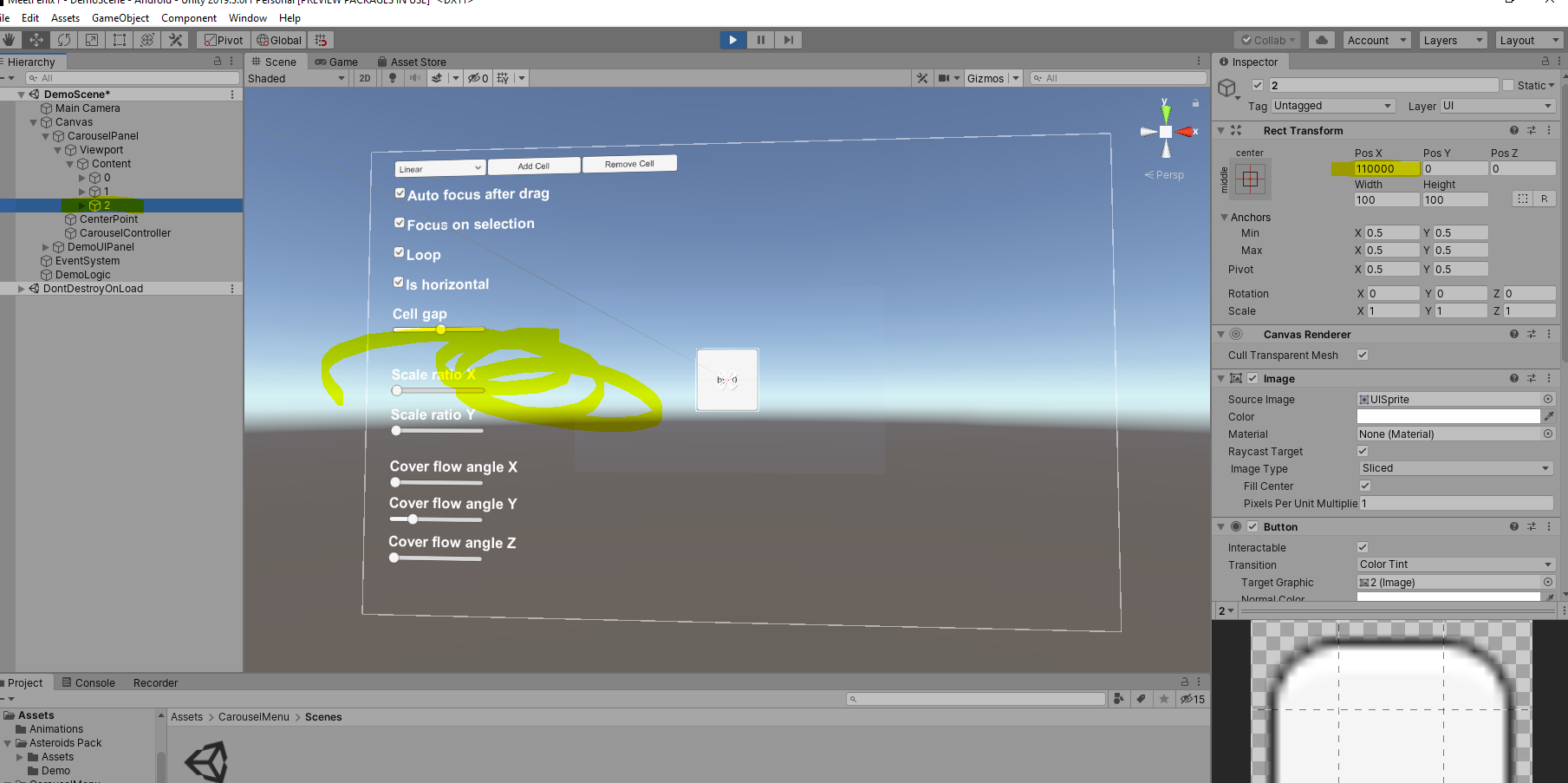Developer

ok thanks for the screenshot, I will check

Hi,

do you know how to fix it?

Developer

hi
Sorry I have been busy with other projects, and thus it may take me awhile to fix these.

But generally speaking, the scale needs to be included in every position update, I believe.

I will find time to update a new version.

Regards,

Developer

while I am checking on this issue, I think one way you can do is to adjust the rect pos Z if you wanna make UI bigger/smaller

Developer

Try this: The code is not properly formatted, but you can search for  _canvasRect.localScale.

only 3 parts are modified to make the logic work in different canvas scale

Let me know if you still have any issues

Thanks

```void SetupCells(bool instantUpdate)
{
for (int i = 0; i < m_cellContainer.Count; i++)
{
RectTransform rt = m_cellContainer[i].GetComponent<recttransform>();
if (rt == null)
continue;
m_newPos = Vector3.zero;
m_newScale = new Vector3(1, 1, 1);
m_newRot = Vector3.zero;
m_offset = (_panel.position - m_dragStartPos);
m_offsetIndex = i - m_centerCellIndex;
m_isCircularMovment = false;
// Consider the canvas scale
m_width = (rt.rect.width + _cellGap) * _canvasRect.localScale.x;
switch (_carouselType)
{
case CarouselConstants.iCarouselType.iCarouselTypeLinear:
{
m_newPos.x = m_width * m_offsetIndex;
}
break;
case CarouselConstants.iCarouselType.iCarouselTypeScaledLinear:
{
m_newPos.x = m_width * m_offsetIndex;
float dis = Vector3.Distance(rt.position, _center.position);
// Make sure it is not too small nor not too big
float scaleRatio = Mathf.Clamp(1 - Mathf.Abs(dis / (_scrollRT.rect.width / 2)), 0.1f, 100);
m_newScale = new Vector3(scaleRatio * _scaleRatio.x, scaleRatio * _scaleRatio.y, scaleRatio * _scaleRatio.z);
}
break;
case CarouselConstants.iCarouselType.iCarouselTypeCoverFlow:
{
m_newPos.x = m_width * m_offsetIndex;
if (m_offsetIndex < 0)
{
m_newRot = -_coverflowAngles;
}
else if (m_offsetIndex > 0)
{
m_newRot = _coverflowAngles;
}
}
break;
case CarouselConstants.iCarouselType.iCarouselTypeScaledCoverFlow:
{
m_newPos.x = m_width * m_offsetIndex;
float dis = Vector3.Distance(rt.position, _center.position);
// Make sure it is not too small nor not too big
float scaleRatio = Mathf.Clamp(1 - Mathf.Abs(dis / (_scrollRT.rect.width / 2)), 0.1f, 100);
m_newScale = new Vector3(scaleRatio * _scaleRatio.x, scaleRatio * _scaleRatio.y, scaleRatio * _scaleRatio.z);
if (m_offsetIndex < 0)
{
m_newRot = -_coverflowAngles;
}
else if (m_offsetIndex > 0)
{
m_newRot = _coverflowAngles;
}
}
break;

}
// Only allow one direction at a time
if (!_isHorizontal)
{
m_newPos.y = m_newPos.x;
m_newPos.x = 0;
m_newRot.x = m_newRot.y;
m_newRot.y = 0;
}
m_final = (_center.position + m_newPos + m_offset);
if (instantUpdate || m_isCircularMovment)
{
rt.position = m_final;
rt.localScale = m_newScale;
rt.localRotation = Quaternion.Euler(m_newRot);
_panel.ForceUpdateRectTransforms();
}
else
{
rt.position = Vector3.Lerp(rt.position, m_final, Time.deltaTime * _moveSpeed);
rt.localScale = Vector3.Lerp(rt.localScale, m_newScale, Time.deltaTime * _scaleSpeed);
rt.localRotation = Quaternion.Lerp(rt.localRotation, Quaternion.Euler(m_newRot), Time.deltaTime * _rotateSpeed);
}
}
}
void CheckBoundary()
{
if (_cell == null)
return;
if (!_shouldLoop)
return;
if (m_cellContainer.Count == 0)
return;
RectTransform rt = _cell.GetComponent<recttransform>();
if (rt == null)
return;
float cellWidth = (rt.rect.width + _cellGap) * _canvasRect.localScale.x;
float cellHeight = (rt.rect.height + _cellGap) * _canvasRect.localScale.y;
// Calculate the boundaries
m_boundary.x = m_cellContainer.Count * cellWidth;
m_boundary.y = m_cellContainer.Count * cellHeight;
float leftBoundary = (_center.position.x - m_boundary.x / 2);
float rightBoundary = (_center.position.x + m_boundary.x / 2);
float upBoundary = (_center.position.y + m_boundary.y / 2);
float downBoundary = (_center.position.y - m_boundary.y / 2);
// We only check the right most or left most
if (_isHorizontal)
{
//if ((_panel.position - m_dragDir).x < 0)
{
GameObject go = m_cellContainer;
if (go.transform.position.x < leftBoundary)
{
//Debug.Log("left");
UpdateBoundaryCell(go, new Vector3(rightBoundary, go.transform.position.y, go.transform.position.z), false);
}
}
//else if ((_panel.position - m_dragDir).x > 0)
{
GameObject go = m_cellContainer[m_cellContainer.Count - 1];
if (go.transform.position.x > rightBoundary)
{
//Debug.Log("right");
UpdateBoundaryCell(go, new Vector3(leftBoundary, go.transform.position.y, go.transform.position.z), true);
}
}
}
else
{
//if ((_panel.position - m_dragDir).y < 0)
{
GameObject go = m_cellContainer;
if (go.transform.position.y < downBoundary)
{
//Debug.Log("down");
UpdateBoundaryCell(go, new Vector3(go.transform.position.x, upBoundary, go.transform.position.z), false);
}
}
//else if ((_panel.position - m_dragDir).y > 0)
{
GameObject go = m_cellContainer[m_cellContainer.Count - 1];
if (go.transform.position.y > upBoundary)
{
//Debug.Log("up");
UpdateBoundaryCell(go, new Vector3(go.transform.position.x, downBoundary, go.transform.position.z), true);
}
}
}
}```

m_width = (rt.rect.width + _cellGap) * _canvasRect.localScale.x;

UnassignedReferenceException: The variable _canvasRect of CarouselController has not been assigned.
You probably need to assign the _canvasRect variable of the CarouselController script in the inspector.
UnityEngine.Transform.get_localScale () (at <7559bf9767e74ff5906f18401f66cd57>:0)

Developer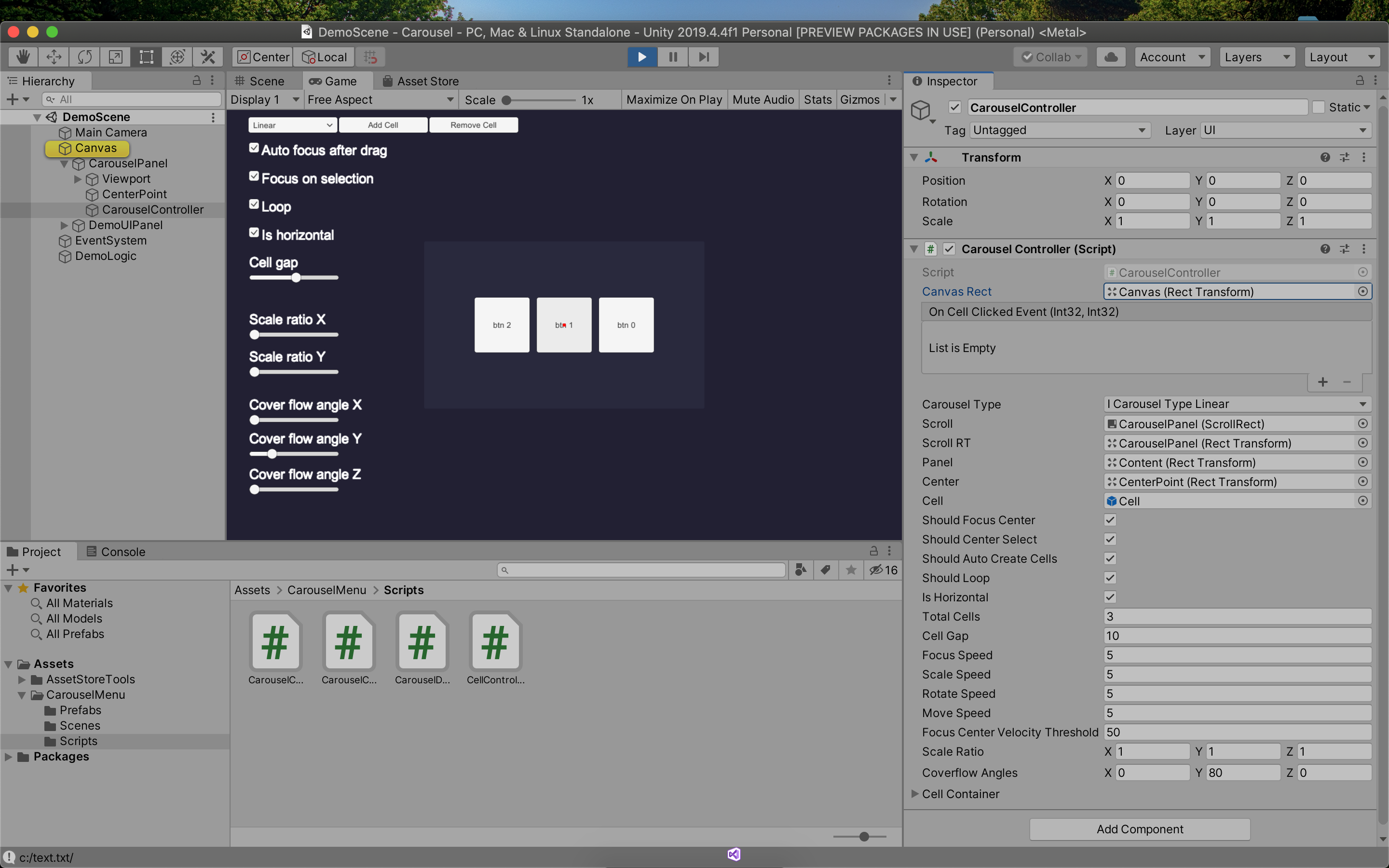there was missing

public RectTransform _canvasRect;

float m_width;

and assign canvas to public field

but still not working, can you update code on unity asset store?